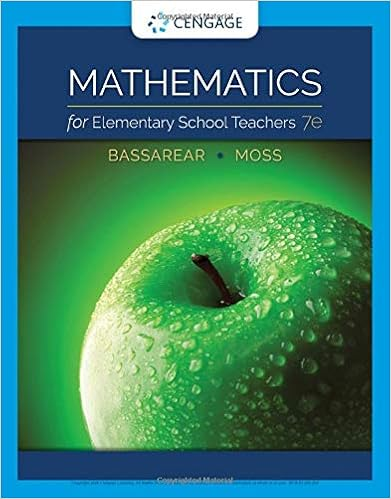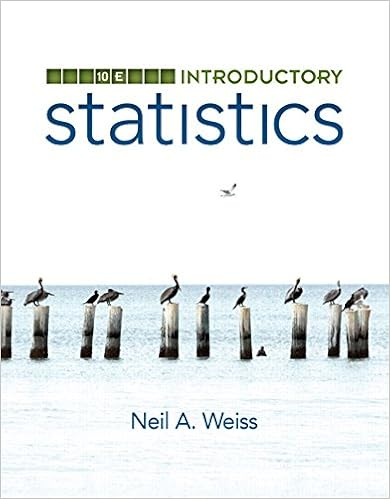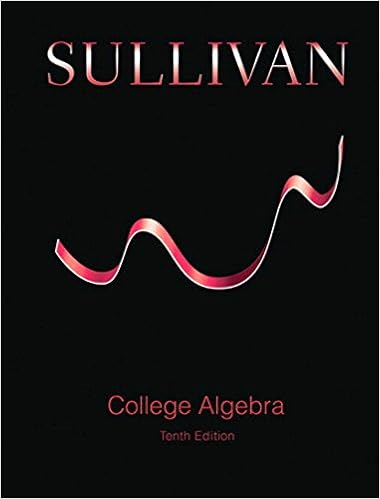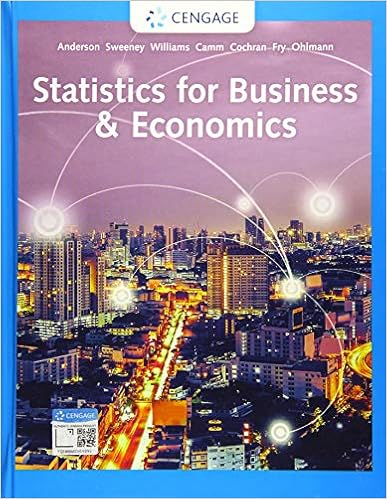# Project 1 Math114.docx - MATH 114 PROJECT 1 The Range Rule...

• Jessiebear345
• 2
• 81% (31) 25 out of 31 people found this document helpful

This preview shows page 1 - 2 out of 2 pages.

The preview shows page 1 - 2 out of 2 pages.
MATH 114PROJECT1The Range Rule of Thumb is based on the insight that the range is often approximately 4 timesas large as the standard deviation. This insight enables you to quickly estimate the standarddeviation of a data set by finding the maximum and minimum data values, using them tocompute the range, and then dividing this value by 4.1.Each of the following 5 data sets has a mean close to 100, and a standard deviation in the20s. Assume that each of these are sample data sets.For each of the 5 data sets given below,use the Excel function “=stdev.s” (or technology) to find the actual sample standard deviation(10 points), compute the estimate given by the Range Rule of Thumb (RROT) Estimate (10points), and compute the percentage error of the estimate (10 points). The formula for theRROT estimate is RROT estimate = Range/4 (where the range = maximum value – minimumvalue), on the assumption that the Standard DeviationRROT estimate.The formula used
##### We have textbook solutions for you!The document you are viewing contains questions related to this textbook.
Chapter 4 / Exercise 56
Mathematics for Elementary School Teachers
BassarearExpert VerifiedBrowse all Textbook Solutions

Course Hero member to access this document

End of preview. Want to read all 2 pages?

Course Hero member to access this document

Term
Summer
Professor
N/A
TagsExpert Verified
•••